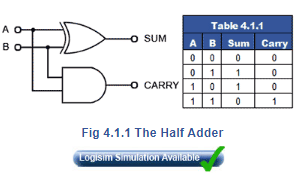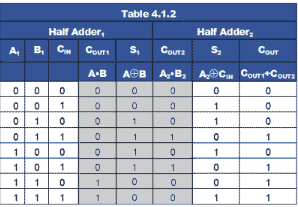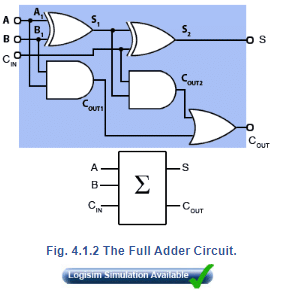# Half Adder & Full Adder Notes | Study Digital Electronics - Electrical Engineering (EE)

Introduction of Half Adder & Full Adder in English is available as part of our Digital Electronics for Electrical Engineering (EE) & Half Adder & Full Adder in Hindi for Digital Electronics course. Download more important topics related with Combinational Logic, notes, lectures and mock test series for Electrical Engineering (EE) Exam by signing up for free. Electrical Engineering (EE): Half Adder & Full Adder Notes | Study Digital Electronics - Electrical Engineering (EE)
 1 Crore+ students have signed up on EduRev. Have you?

Binary Arithmetic  is carried out by combinational logic circuits, the simplest of which is the half adder, shown in Fig. 4.1.1. This circuit consists, in its most basic form of two gates, an XOR gate  that produces a logic 1 output whenever A is 1 and B is 0, or when B is 1 and A is 0. The AND gate produces a logic 1 at the carry output when both A and B are 1. The half adder truth table is shown in Table 4.1.1 and describes the result of binary addition.1 plus 0 = 12 (110)

and

1 plus 1 = 102 (210)

The half adder is fine for adding two 1-bit numbers together, but for binary numbers containing several bits, a carry may be produced at some time (as a result of adding 1 and 1) that must be added to the next column. As the half adder has only two inputs it cannot add in a carry bit from a previous column, so it is not practical for anything other than 1-bit additions.When 2 or more bits are to be added, the circuit used is the Full Adder, shown in Fig 4.1.2, (blue background) together with its simplified block diagram symbol. This circuit simply comprises two half adders, the sum of A and B from the first half adder is used as input A on the second half adder, which now produces a sum of the first half adder sum (S1) plus any ‘carry in’ from the CIN terminal. Any carries produced by the two half adders are then ‘ORed’ together to produce a single COUT. output. The truth table for the circuit is given in Table 4.1.2.The document Half Adder & Full Adder Notes | Study Digital Electronics - Electrical Engineering (EE) is a part of the Electrical Engineering (EE) Course Digital Electronics.
All you need of Electrical Engineering (EE) at this link: Electrical Engineering (EE)

## Digital Electronics

88 videos|71 docs|8 tests
 Use Code STAYHOME200 and get INR 200 additional OFF

## Digital Electronics

88 videos|71 docs|8 tests

Track your progress, build streaks, highlight & save important lessons and more!

,

,

,

,

,

,

,

,

,

,

,

,

,

,

,

,

,

,

,

,

,

;